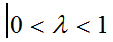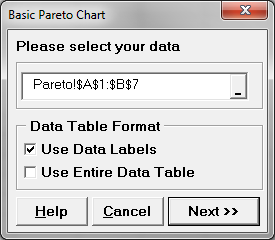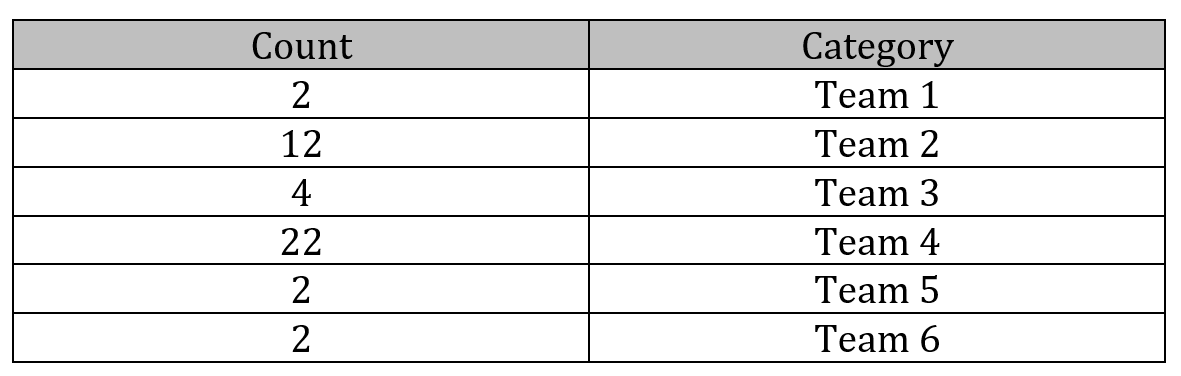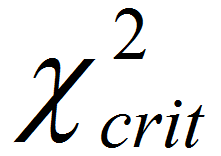Top NavEWMA Chart with Minitab

What is an EWMA Chart? The EWMA chart (Exponentially-Weighted Moving Average Chart) is a control chart monitoring the exponentially-weighted average of previous and present subgroup means. The more recent data get more weight than older data. It detects the shift of the process mean from the process target over time. The underlying distribution of the [...]Pareto Analysis with SigmaXL

Pareto Principle The Pareto principle is an observation not a law.  Named after Italian economist Vilfredo Pareto, this principle states that for a variety of situations, 80% of consequences come from 20% of the causes, thus the Pareto principle is also known as the 80/20 rule. The 80/20 rule basically reminds us that the comparison of [...]Pareto Analysis with Minitab

What is the Pareto Principle? The Pareto principle is an observation not a law.  Named after Italian economist Vilfredo Pareto, this principle states that for a variety of situations, 80% of consequences come from 20% of the causes, thus the Pareto principle is also known as the 80/20 rule. The 80/20 rule basically reminds us that [...]Moods Median Test with JMP

What is Moods Median Test? Mood’s median test is a statistical test to compare the medians of two or more populations. Null Hypothesis (H0): η1 = … = ηk Alternative Hypothesis (Ha): At least one of the medians is different from the others The symbol k is the number of groups of our interest and [...]Pareto Analysis with JMP

What is the Pareto Principle? The Pareto principle is an observation not a law.  Named after Italian economist Vilfredo Pareto, this principle states that for a variety of situations, 80% of consequences come from 20% of the causes, thus the Pareto principle is also known as the 80/20 rule. The 80/20 rule basically reminds us that [...]Moods Median Test with SigmaXL

What is Mood’s Median Test? Mood’s median test is a statistical test to compare the medians of two or more populations. Null Hypothesis (H0): η1 = … = ηk Alternative Hypothesis (Ha): At least one of the medians is different from the others The symbol k is the number of groups of our interest and [...]Moods Median Test with Minitab

What is Mood’s Median Test? Mood’s median test is a statistical test to compare the medians of two or more populations. Null Hypothesis (H0): η1 = … = ηk Alternative Hypothesis (Ha): At least one of the medians is different from the others The symbol k is the number of groups of our interest and [...]